﻿ Northlake Preschool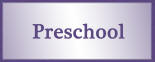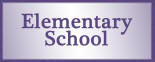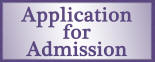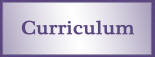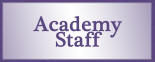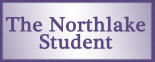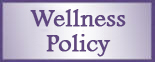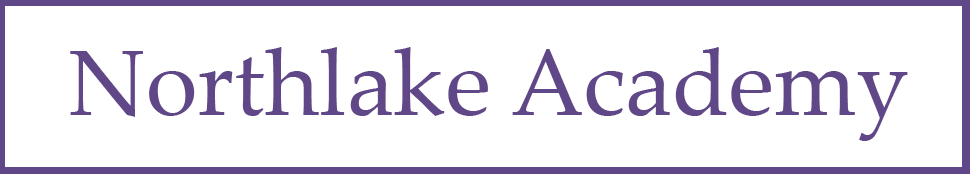Number and Numeration

 Program Goal Content Thread Grade-Level Goal Understand the Meanings, Uses, and Representations of Numbers Place value and notation Read and write whole numbers up to 1,000,000; read, write, and model with manipulatives decimals through hundredths; identify places in such numbers and the values of the digits in those places; translate between whole numbers and decimals represented in words, in base-10 notation, and with manipulatives. Meanings and uses of fractions Read, write, and model fractions; solve problems involving fractional parts of a region or a collection; describe strategies used. Number theory Find multiples of 2, 5, and 10. Understand Equivalent Names for Numbers Equivalent names for whole numbers Use numerical expressions involving one or more of the basic four arithmetic operations to give equivalent names for whole numbers. Equivalent names for fractions, decimals, and percents Use manipulatives and drawings to find and represent equivalent names for fractions; use manipulatives to generate equivalent fractions. Understand Common Numerical Relations Comparing and ordering numbers Compare and order whole numbers up to 1,000,000; use manipulatives to order decimals through hundredths; use area models and benchmark fractions to compare and order fractions.

Operations and Computation

 Program Goal Content Thread Grade-Level Goal Compute Accurately Addition and subtraction facts Demonstrate automaticity with all addition and subtraction facts through 10+10; use basic facts to compute fact extensions such as 80+70. Addition and subtraction procedures Use manipulatives, mental arithmetic, paper-and-pencil algorithms and models, and calculators to solve problems involving the addition and subtraction of whole numbers and decimals in a money context; describe the strategies used and explain how they work. Multiplication and division facts Demonstrate automaticity with multiplication facts through 10 x 10. Multiplication and division procedures Use arrays, mental arithmetic, paper-and-pencil algorithms and models, and calculators to solve problems involving the multiplication of 2- and 3-digit whole numbers by 1-digit whole numbers; describe the strategies used. Make Reasonable Estimates Computational estimation Make reasonable estimates for whole number addition, subtraction, multiplication, and division problems; explain how the estimates were obtained. Understand Meanings of Operations Models for the operations Recognize and describe change, comparison, and parts-and-total situations; use repeated addition, arrays, and skip counting to model multiplication; use equal sharing and equal grouping to model division.

Data and Chance

 Program Goal Content Thread Grade-Level Goal Select and Create Appropriate Graphical Representations of Collected or Given Data Data collection and representation Collect and organize data or use given data to create charts, tables, graphs, and line plots. Analyze and Interpret Data Data analysis Use graphs to ask and answer simple questions and draw conclusions; find the maximum, minimum, range, mode, and median of a data set. Understand and Apply Basic Concepts of Probability Qualitative probability Describe events using certain, very likely, likely, unlikely, very unlikely, impossible and other basic probability terms; explain the choice of language. Quantitative probability Predict the outcomes of simple experiments and test the predictions using manipulatives; express the probability of an event by using "_out of _" language.

Measurement and Reference Frames

 Program Goal Content Thread Grade-Level Goal Understand the Systems and Processes of Measurement; Use Appropriate Techniques, Tools, Units, and Formulas in Making Measurements Length, weight, and angles Estimate length with and without tools; measure length to the nearest 1/2 inch and 1/2 centimeter; draw and describe angles as records of rotations. Area, perimeter, volume, and capacity Describe and use strategies to measure the perimeter of polygons; find the areas of rectangles. Units and systems of measurement Describe relationships among inches, feet, and yards; describe relationships between minutes in an hour, hours in a day, days in a week. Use and Understand Reference Frames Time Tell and show time to the nearest minute on an analog clock; tell and write time in digital notation.

Geometry

 Program Goal Content Thread Grade-Level Goal Investigate Characteristics and Properties of Two- and Three-Dimensional Geometric Shapes Lines and angles Identify and draw points, intersecting and parallel line segments and lines, rays, and right angles. Plane and solid figures Identify, describe, model, and compare plane and solid figures including circles, polygons, spheres, cylinders, rectangular prisms, pyramids, cones, and cubes using appropriate geometric terms including the terms face, edge, vertex, and base. Apply Transformations and Symmetry in Geometric Situations Transformations and symmetry Create and complete two-dimensional symmetric shapes or designs; locate multiple lines of symmetry in a two-dimensional shape.

Patterns, Functions, and Algebra

 Program Goal Content Thread Grade-Level Goal Understand Patterns and Functions Patterns and functions Extend, describe, and create numeric patterns; describe rules for patterns and use them to solve problems; use words and symbols to describe and write rules for functions involving addition, subtraction, and multiplication and use those rules to solve problems. Use Algebraic Notation to Represent and Analyze Situations and Structures Algebraic notation and solving number sentences Read, write, and explain number sentences using the symbols +,-,x, Ë†, =, >, and <; solve number sentences; write expressions and number sentences to model number stories. Order of operations Recognize that numeric expressions can have different values depending on the order in which operations are carried out; understand that grouping symbols can be used to affect the order in which operations are carried out. Properties of the arithmetic operations Describe and apply the Commutative and Associative Properties of Addition and Multiplication and the Multiplicative Identity; apply the Distributive Property of Multiplication over Addition.